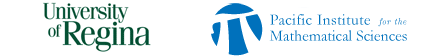SEARCH HOMEMath Central Quandaries & QueriesQuestion from Samantha, a student: Is the square of an odd number always odd?We have two responses for you.

Hi Samantha. Let's look at a couple of small odd numbers first:

12 = 1, and that's clearly odd.

32 = 9, which is odd. We can write a table like this:

32 =
 1 2 31 1 2 3 2 4 5 6 3 7 8 9

green is for odd and purple is for even. So it continues...

52 =
 1 2 3 4 51 1 2 3 4 5 2 6 7 8 9 10 3 11 12 13 14 15 4 16 17 18 19 20 5 21 22 23 24 25

72 =
 1 2 3 4 5 6 71 1 2 3 4 5 6 7 2 8 9 10 11 12 13 14 3 15 16 17 18 19 20 21 4 22 23 24 25 26 27 28 5 29 30 31 32 33 34 35 6 36 37 38 39 40 41 42 7 43 44 45 46 47 48 49

In each row, whatever colour starts the row is the colour that ends the row. Since the diagram is a square, then there are an odd number of rows, too. That means whatever colour is at the top of a column is also at the bottom of a column. Since all the tables begin with 1, which is odd and therefore green, we can choose any arbitrary odd number and write its square table and we will get all the corners as odd.

odd2 =
 1 2 ... ... ... ... odd1 odd even . even odd 2 even odd . odd even . . . ... ... ... . . . even odd . odd even odd odd even . even odd

And you can see that this means the bottom right hand corner, which is the value of odd2 , is also going to be odd.

Stephen La Rocque.>

Hi Samantha,

If you know some algebra you can try it this way. An odd number is one more than an even number so you can write your odd number as 2n + 1 where n is an integer. Then the square of this number is

(2n + 1)(2n + 1)

Expand this to get

(2n + 1)(2n + 1) = 4n2 + 4n + 1 = 2(2n2 + 2n) + 1

which is again one more than an even number so it is odd.

Penny* Registered trade mark of Imperial Oil Limited. Used under license.Math Central is supported by the University of Regina and the Imperial Oil Foundation.